# NCERT Exemplar Class 11 Chemistry Solutions for Chapter 9 - Hydrogen

## NCERT Exemplar Solutions Class 11 Chemistry Chapter 9 – Free PDF Download

NCERT Exemplar Chemistry Class 11 Chapter 9 Hydrogen is given here to help you get better equipped for CBSE Class 11 Chemistry exam and competitive entrance examinations like NEET, IIT-JEE, AIEE. These solutions are the best tool that can help you in understanding the topic clearly. NCERT Exemplar Class 11 Chemistry Chapter 9 PDF presents you a variety of questions like MCQs, match the following, filling in the blanks, numerical problems, exercises, worksheets, compound nomenclature and so on.

Chapter 9 of NCERT Exemplar Class 11 Chemistry was designed to teach students about hydrogen its properties, its preparation. After reading the chapter students will learn how different elements combine with hydrogen to form ionic, molecular and nonstoichiometric compounds. Students will also learn some important terms like

• Electron-deficient
• Electron precise,
• Electron-rich
• Hydrogen economy
• Hydrogenation

### Download PDF of NCERT Exemplar Solutions For Class 11 Chemistry Chapter 9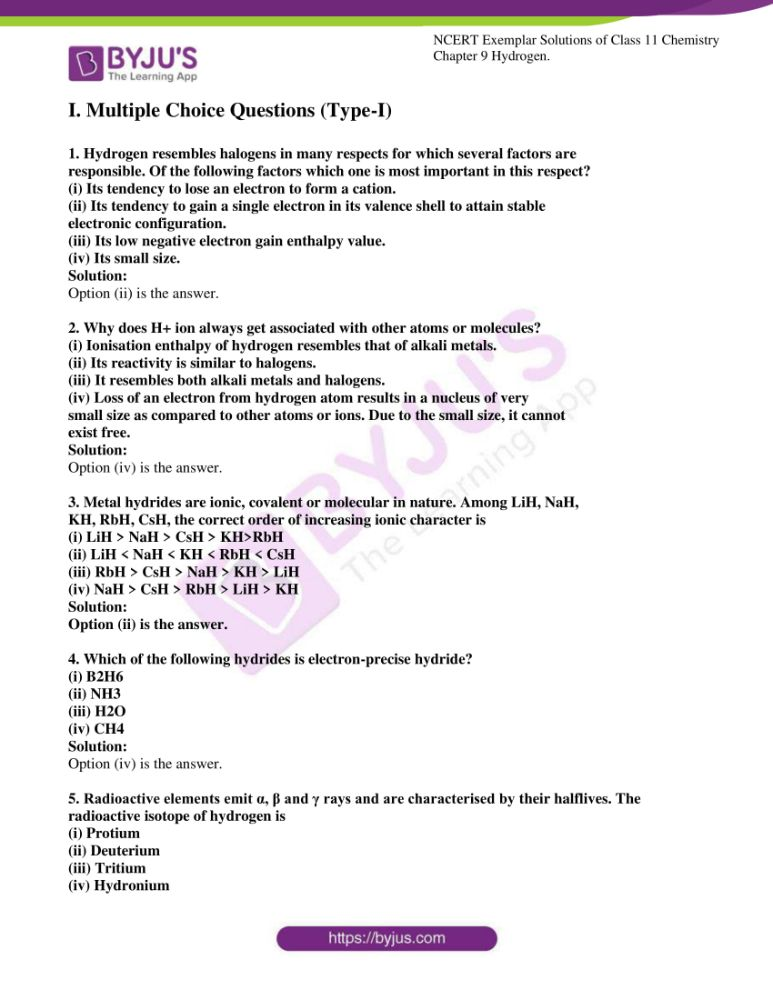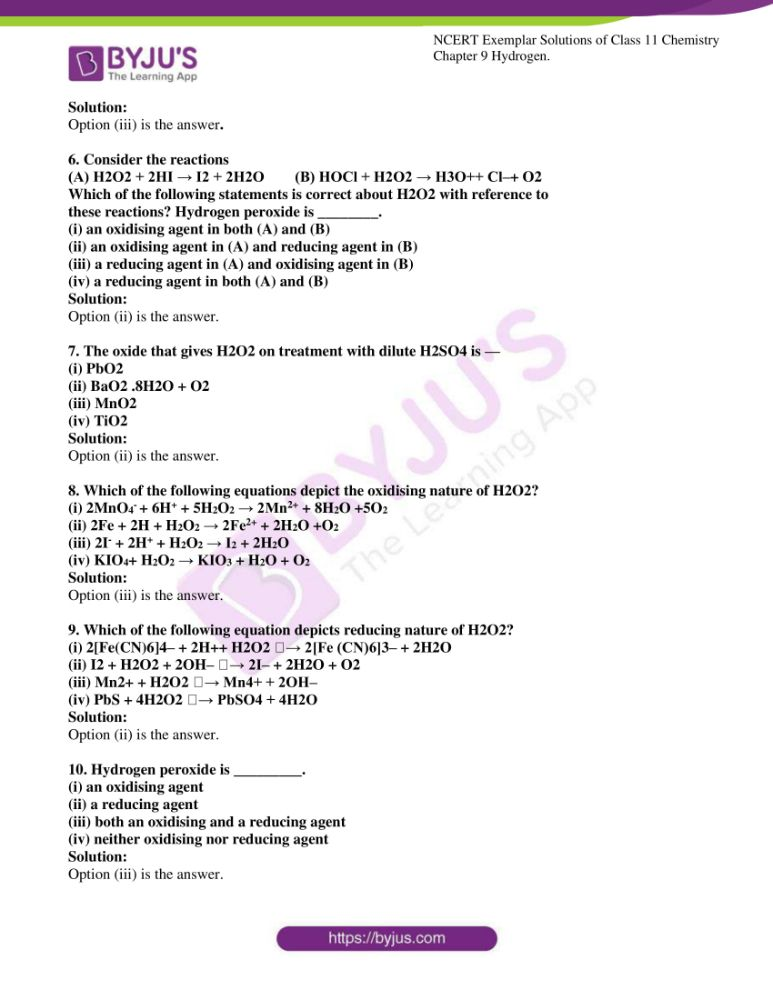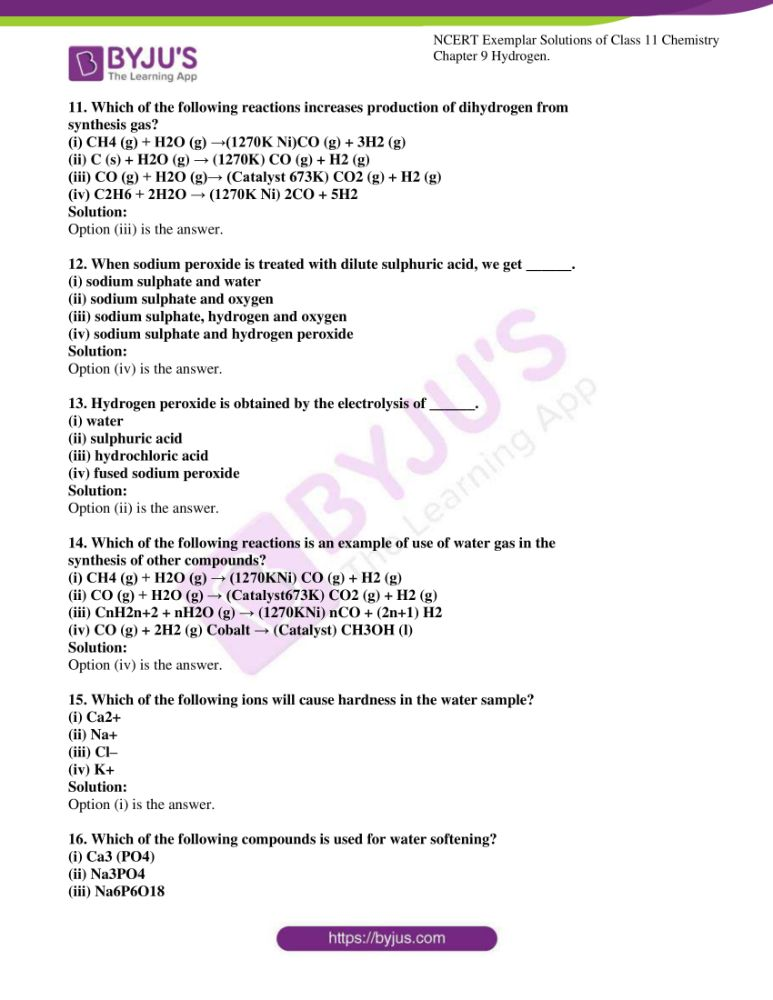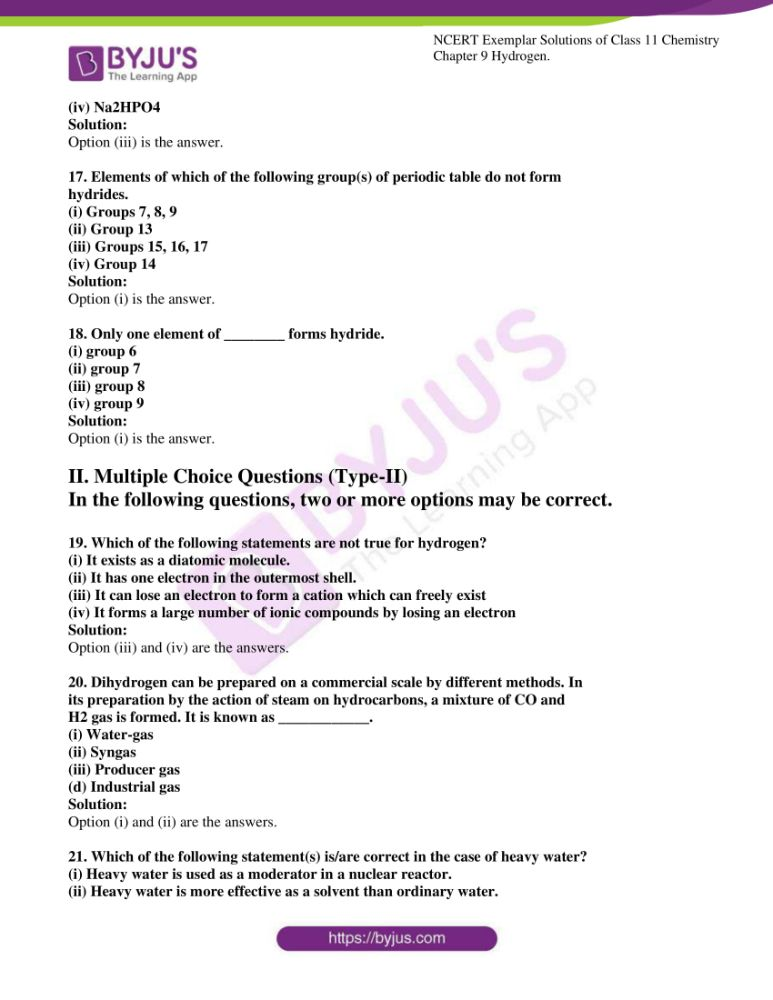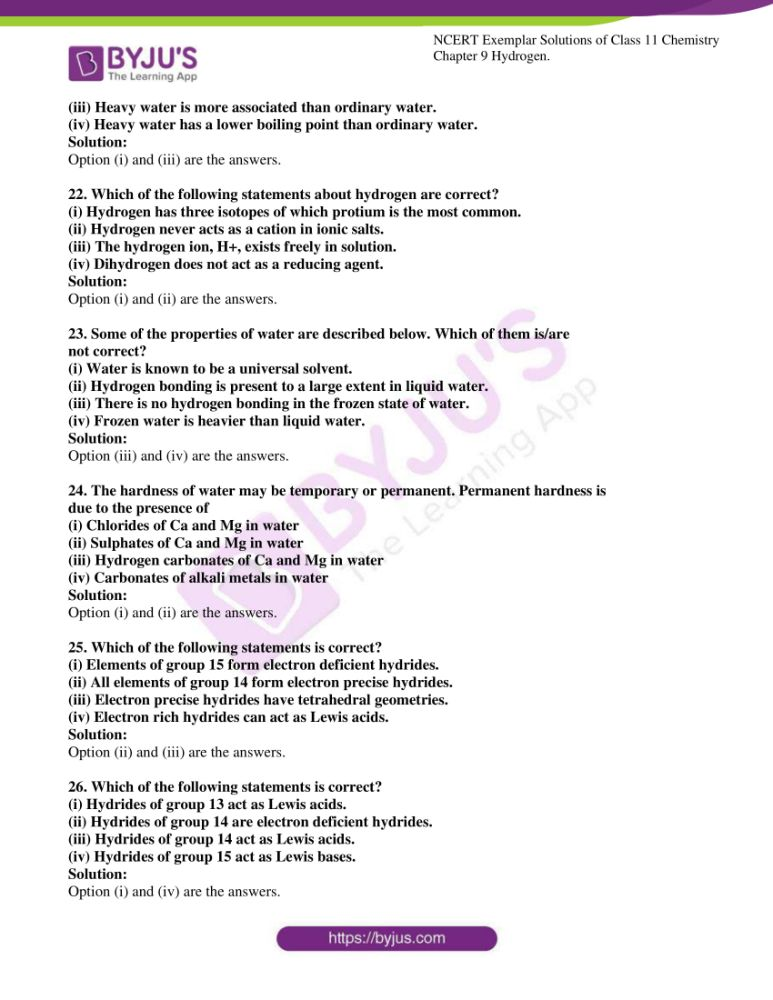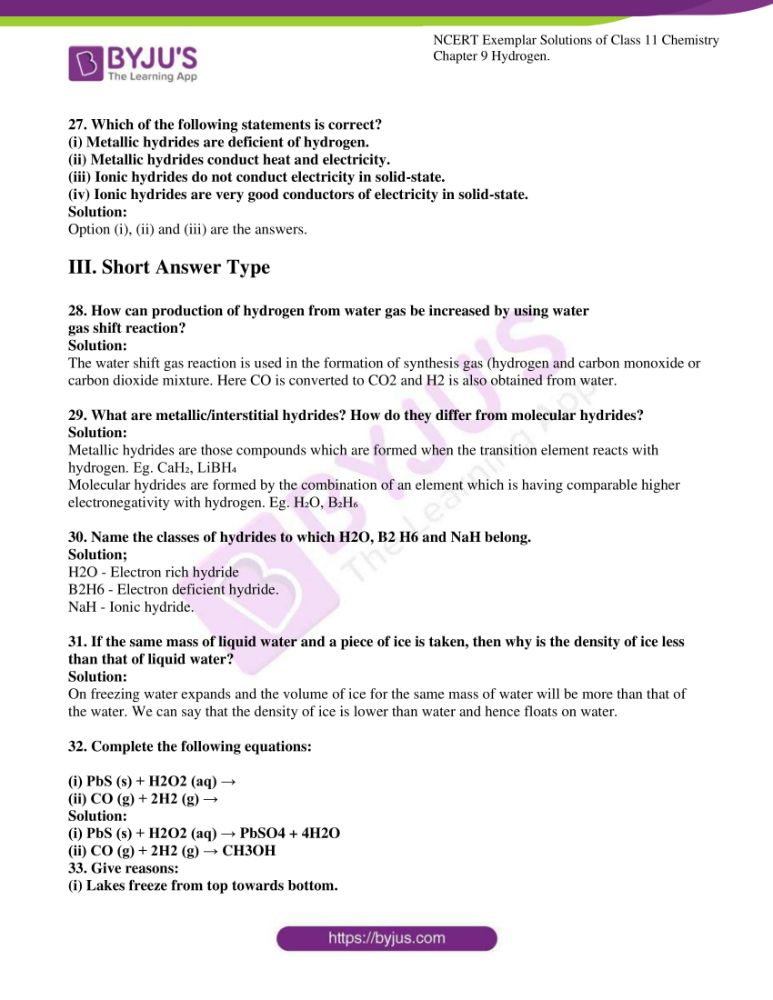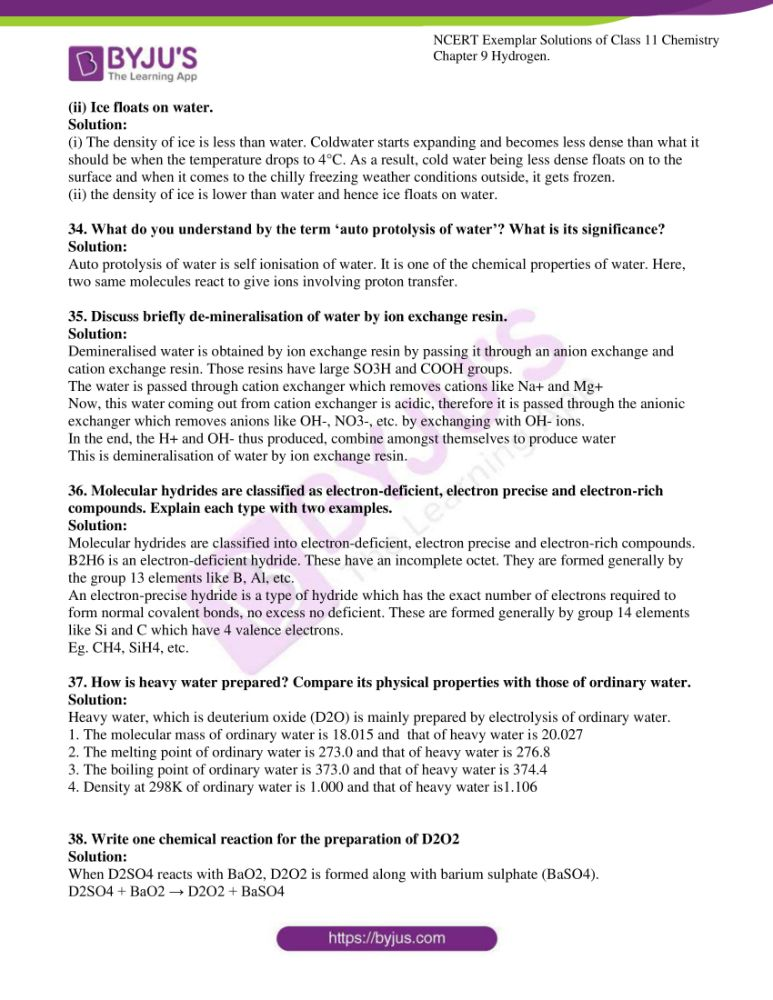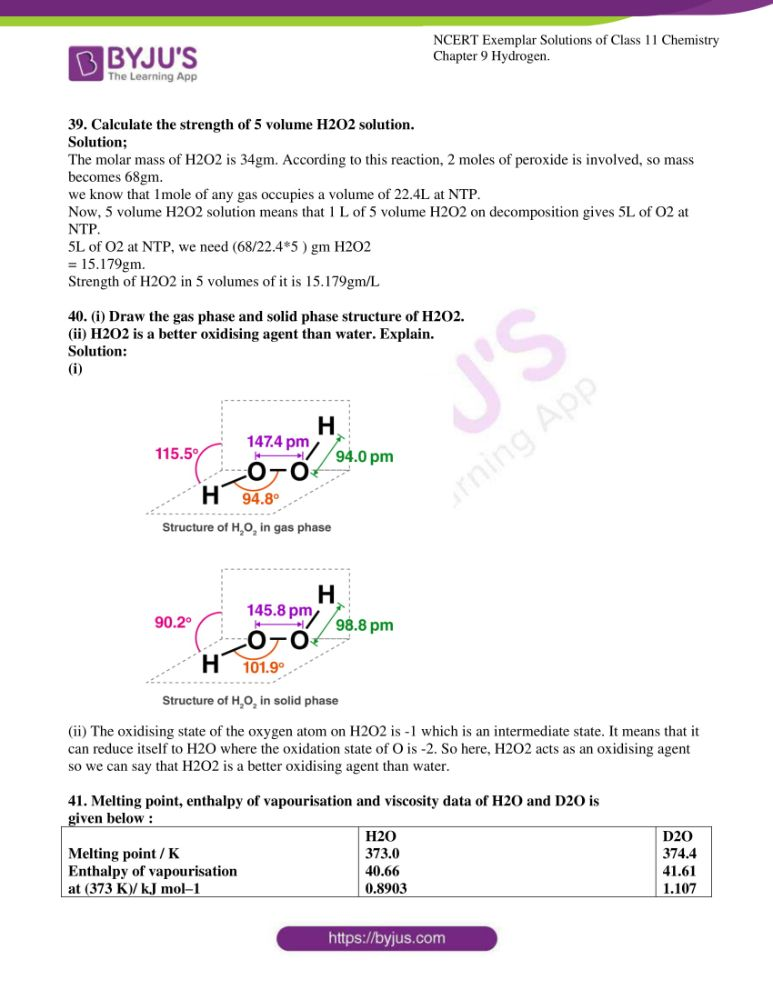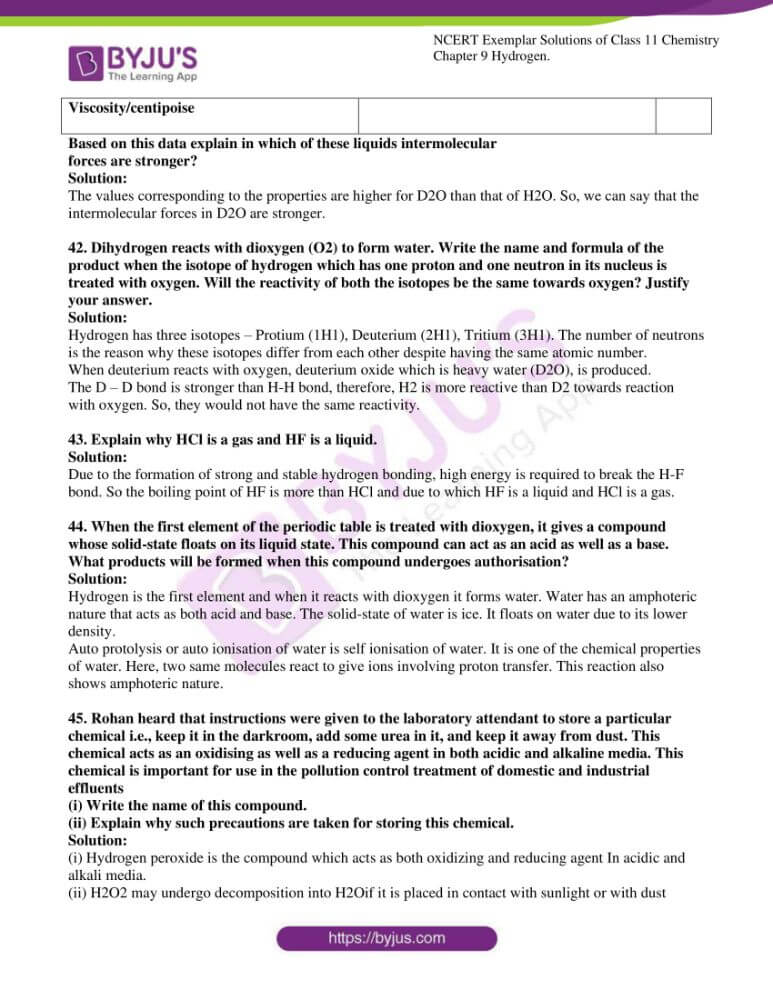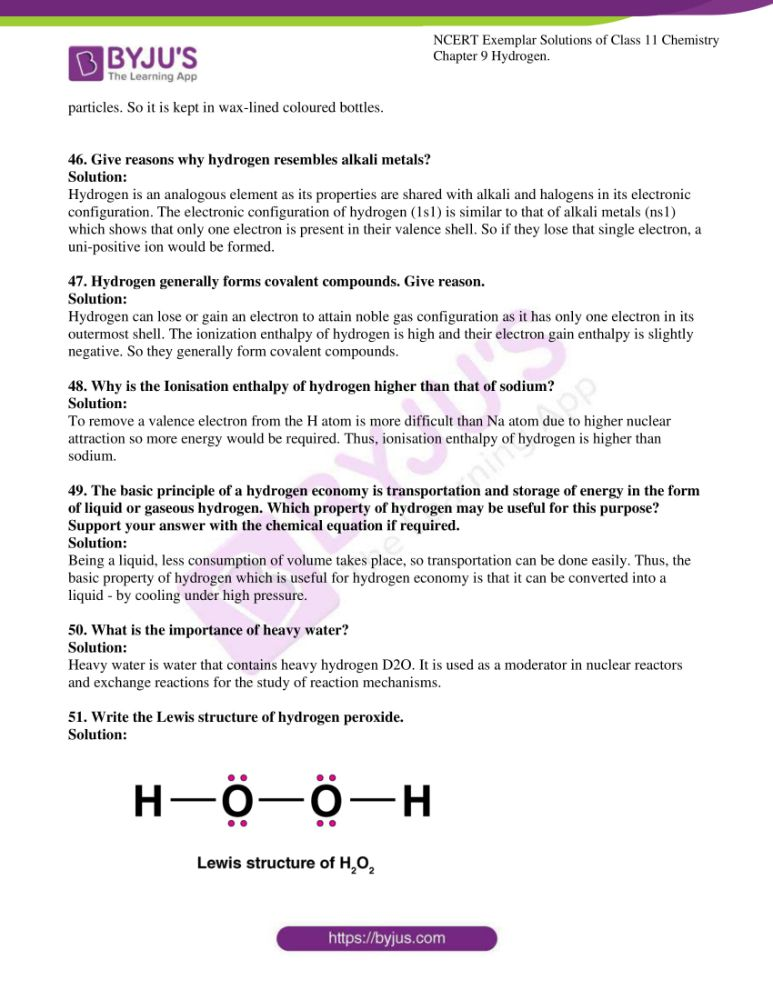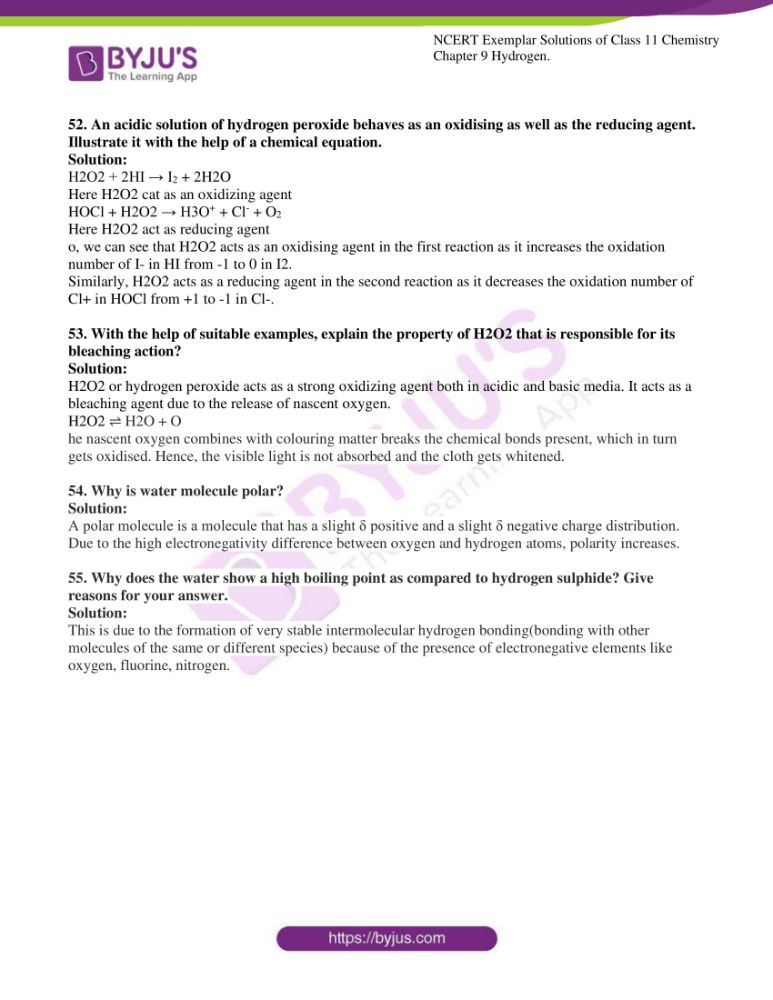### Access NCERT Exemplar Solutions For Class 11 Chemistry Chapter 9

I. Multiple Choice Questions (Type-I)

1. Hydrogen resembles halogens in many respects for which several factors are

responsible. Of the following factors which one is most important in this respect?

(i) Its tendency to lose an electron to form a cation.

(ii) Its tendency to gain a single electron in its valence shell to attain stable

electronic configuration.

(iii) Its low negative electron gain enthalpy value.

(iv) Its small size.

Solution:

2. Why does H+ ion always get associated with other atoms or molecules?

(i) Ionisation enthalpy of hydrogen resembles that of alkali metals.

(ii) Its reactivity is similar to halogens.

(iii) It resembles both alkali metals and halogens.

(iv) Loss of an electron from hydrogen atom results in a nucleus of very

small size as compared to other atoms or ions. Due to the small size, it cannot

exist free.

Solution:

3. Metal hydrides are ionic, covalent or molecular in nature. Among LiH, NaH,

KH, RbH, CsH, the correct order of increasing ionic character is

(i) LiH > NaH > CsH > KH>RbH

(ii) LiH < NaH < KH < RbH < CsH

(iii) RbH > CsH > NaH > KH > LiH

(iv) NaH > CsH > RbH > LiH > KH

Solution:

4. Which of the following hydrides is electron-precise hydride?

(i) B2H6

(ii) NH3

(iii) H2O

(iv) CH4

Solution:

5. Radioactive elements emit α, β and γ rays and are characterised by their halflives. The radioactive isotope of hydrogen is

(i) Protium

(ii) Deuterium

(iii) Tritium

(iv) Hydronium

Solution:

6. Consider the reactions

(A) H2O2 + 2HI → I2 + 2H2O (B) HOCl + H2O2 → H3O++ Cl–+ O2

Which of the following statements is correct about H2O2 with reference to

these reactions? Hydrogen peroxide is ________.

(i) an oxidising agent in both (A) and (B)

(ii) an oxidising agent in (A) and reducing agent in (B)

(iii) a reducing agent in (A) and oxidising agent in (B)

(iv) a reducing agent in both (A) and (B)

Solution:

7. The oxide that gives H2O2 on treatment with dilute H2SO4 is —

(i) PbO2

(ii) BaO2 .8H2O + O2

(iii) MnO2

(iv) TiO2

Solution:

8. Which of the following equations depict the oxidising nature of H2O2?

(i) 2MnO4+ 6H+ + 5H2O2 → 2Mn2+ + 8H2O +5O2

(ii) 2Fe + 2H + H2O2 → 2Fe2+ + 2H2O +O2

(iii) 2I + 2H+ + H2O2 → I2 + 2H2O

(iv) KIO4+ H2O2 → KIO3 + H2O + O2

Solution:

9. Which of the following equation depicts reducing nature of H2O2?

(i) 2[Fe(CN)6]4– + 2H++ H2O2 → 2[Fe (CN)6]3– + 2H2O

(ii) I2 + H2O2 + 2OH– → 2I– + 2H2O + O2

(iii) Mn2+ + H2O2 → Mn4+ + 2OH–

(iv) PbS + 4H2O2 → PbSO4 + 4H2O

Solution:

10. Hydrogen peroxide is _________.

(i) an oxidising agent

(ii) a reducing agent

(iii) both an oxidising and a reducing agent

(iv) neither oxidising nor reducing agent

Solution:

11. Which of the following reactions increases production of dihydrogen from

synthesis gas?(i) CH4 (g) + H2O (g) →(1270K Ni)CO (g) + 3H2 (g)

(ii) C (s) + H2O (g) → (1270K) CO (g) + H2 (g)

(iii) CO (g) + H2O (g)→ (Catalyst 673K) CO2 (g) + H2 (g)

(iv) C2H6 + 2H2O → (1270K Ni) 2CO + 5H2

Solution:

12. When sodium peroxide is treated with dilute sulphuric acid, we get ______.

(i) sodium sulphate and water

(ii) sodium sulphate and oxygen

(iii) sodium sulphate, hydrogen and oxygen

(iv) sodium sulphate and hydrogen peroxide

Solution:

13. Hydrogen peroxide is obtained by the electrolysis of ______.

(i) water

(ii) sulphuric acid

(iii) hydrochloric acid

(iv) fused sodium peroxide

Solution:

14. Which of the following reactions is an example of use of water gas in the

synthesis of other compounds?

(i) CH4 (g) + H2O (g) → (1270KNi) CO (g) + H2 (g)

(ii) CO (g) + H2O (g) → (Catalyst673K) CO2 (g) + H2 (g)

(iii) CnH2n+2 + nH2O (g) → (1270KNi) nCO + (2n+1) H2

(iv) CO (g) + 2H2 (g) Cobalt → (Catalyst) CH3OH (l)

Solution:

15. Which of the following ions will cause hardness in the water sample?

(i) Ca2+

(ii) Na+

(iii) Cl–

(iv) K+

Solution:

16. Which of the following compounds is used for water softening?

(i) Ca3 (PO4)

(ii) Na3PO4

(iii) Na6P6O18

(iv) Na2HPO4

Solution:

17. Elements of which of the following group(s) of periodic table do not form

hydrides.

(i) Groups 7, 8, 9

(ii) Group 13

(iii) Groups 15, 16, 17

(iv) Group 14

Solution:

18. Only one element of ________ forms hydride.

(i) group 6

(ii) group 7

(iii) group 8

(iv) group 9

Solution:

II. Multiple Choice Questions (Type-II)

In the following questions, two or more options may be correct.

19. Which of the following statements are not true for hydrogen?

(i) It exists as a diatomic molecule.

(ii) It has one electron in the outermost shell.

(iii) It can lose an electron to form a cation which can freely exist

(iv) It forms a large number of ionic compounds by losing an electron

Solution:

Option (iii) and (iv) are the answers.

20. Dihydrogen can be prepared on a commercial scale by different methods. In

its preparation by the action of steam on hydrocarbons, a mixture of CO and

H2 gas is formed. It is known as ____________.

(i) Water-gas

(ii) Syngas

(iii) Producer gas

(d) Industrial gas

Solution:

Option (i) and (ii) are the answers.

21. Which of the following statement(s) is/are correct in the case of heavy water?

(i) Heavy water is used as a moderator in a nuclear reactor.

(ii) Heavy water is more effective as a solvent than ordinary water.

(iii) Heavy water is more associated than ordinary water.

(iv) Heavy water has a lower boiling point than ordinary water.

Solution:

Option (i) and (iii) are the answers.

22. Which of the following statements about hydrogen are correct?

(i) Hydrogen has three isotopes of which protium is the most common.

(ii) Hydrogen never acts as a cation in ionic salts.

(iii) The hydrogen ion, H+, exists freely in solution.

(iv) Dihydrogen does not act as a reducing agent.

Solution:

Option (i) and (ii) are the answers.

23. Some of the properties of water are described below. Which of them is/are

not correct?

(i) Water is known to be a universal solvent.

(ii) Hydrogen bonding is present to a large extent in liquid water.

(iii) There is no hydrogen bonding in the frozen state of water.

(iv) Frozen water is heavier than liquid water.Solution:

Option (iii) and (iv) are the answers.

24. The hardness of water may be temporary or permanent. Permanent hardness is

due to the presence of

(i) Chlorides of Ca and Mg in water

(ii) Sulphates of Ca and Mg in water

(iii) Hydrogen carbonates of Ca and Mg in water

(iv) Carbonates of alkali metals in water

Solution:

Option (i) and (ii) are the answers.

25. Which of the following statements is correct?

(i) Elements of group 15 form electron deficient hydrides.

(ii) All elements of group 14 form electron precise hydrides.

(iii) Electron precise hydrides have tetrahedral geometries.

(iv) Electron rich hydrides can act as Lewis acids.

Solution:

Option (ii) and (iii) are the answers.

26. Which of the following statements is correct?

(i) Hydrides of group 13 act as Lewis acids.

(ii) Hydrides of group 14 are electron deficient hydrides.

(iii) Hydrides of group 14 act as Lewis acids.

(iv) Hydrides of group 15 act as Lewis bases.

Solution:

Option (i) and (iv) are the answers.

27. Which of the following statements is correct?

(i) Metallic hydrides are deficient of hydrogen.

(ii) Metallic hydrides conduct heat and electricity.

(iii) Ionic hydrides do not conduct electricity in solid-state.

(iv) Ionic hydrides are very good conductors of electricity in solid-state.

Solution:

Option (i), (ii) and (iii) are the answers.

28. How can production of hydrogen from water gas be increased by using water

gas shift reaction?

Solution:

The water shift gas reaction is used in the formation of synthesis gas (hydrogen and carbon monoxide or carbon dioxide mixture. Here CO is converted to CO2 and H2 is also obtained from water.

29. What are metallic/interstitial hydrides? How do they differ from molecular hydrides?

Solution:

Metallic hydrides are those compounds which are formed when the transition element reacts with hydrogen. Eg. CaH₂, LiBH₄

Molecular hydrides are formed by the combination of an element which is having comparable higher electronegativity with hydrogen. Eg. H₂O, B₂H₆

30. Name the classes of hydrides to which H2O, B2 H6 and NaH belong.

Solution;

H2O – Electron rich hydride

B2H6 – Electron deficient hydride.

NaH – Ionic hydride.

31. If the same mass of liquid water and a piece of ice is taken, then why is the density of ice less than that of liquid water?

Solution:

On freezing water expands and the volume of ice for the same mass of water will be more than that of the water. We can say that the density of ice is lower than water and hence floats on water.

32. Complete the following equations:

(i) PbS (s) + H2O2 (aq) →

(ii) CO (g) + 2H2 (g) →

Solution:

(i) PbS (s) + H2O2 (aq) → PbSO4 + 4H2O

(ii) CO (g) + 2H2 (g) → CH3OH

33. Give reasons:

(i) Lakes freeze from top towards bottom.

(ii) Ice floats on water.

Solution:

(i) The density of ice is less than water. Coldwater starts expanding and becomes less dense than what it should be when the temperature drops to 4°C. As a result, cold water being less dense floats on to the surface and when it comes to the chilly freezing weather conditions outside, it gets frozen.

(ii) the density of ice is lower than water and hence ice floats on water.

34. What do you understand by the term ‘auto protolysis of water’? What is its significance?

Solution:

Auto protolysis of water is self ionisation of water. It is one of the chemical properties of water. Here, two same molecules react to give ions involving proton transfer.

35. Discuss briefly de-mineralisation of water by ion exchange resin.

Solution:

Demineralised water is obtained by ion exchange resin by passing it through an anion exchange and cation exchange resin. Those resins have large SO3H and COOH groups.

The water is passed through cation exchanger which removes cations like Na+ and Mg+

Now, this water coming out from cation exchanger is acidic, therefore it is passed through the anionic exchanger which removes anions like OH-, NO3-, etc. by exchanging with OH- ions.

In the end, the H+ and OH- thus produced, combine amongst themselves to produce water

This is demineralisation of water by ion exchange resin.

36. Molecular hydrides are classified as electron-deficient, electron precise and electron-rich compounds. Explain each type with two examples.

Solution:

Molecular hydrides are classified into electron-deficient, electron precise and electron-rich compounds.

B2H6 is an electron-deficient hydride. These have an incomplete octet. They are formed generally by the group 13 elements like B, Al, etc.

An electron-precise hydride is a type of hydride which has the exact number of electrons required to form normal covalent bonds, no excess no deficient. These are formed generally by group 14 elements like Si and C which have 4 valence electrons.

Eg. CH4, SiH4, etc.

37. How is heavy water prepared? Compare its physical properties with those of ordinary water.

Solution:

Heavy water, which is deuterium oxide (D2O) is mainly prepared by electrolysis of ordinary water.

1. The molecular mass of ordinary water is 18.015 and that of heavy water is 20.027

2. The melting point of ordinary water is 273.0 and that of heavy water is 276.8

3. The boiling point of ordinary water is 373.0 and that of heavy water is 374.4

4. Density at 298K of ordinary water is 1.000 and that of heavy water is1.106

38. Write one chemical reaction for the preparation of D2O2

Solution:

When D2SO4 reacts with BaO2, D2O2 is formed along with barium sulphate (BaSO4).

D2SO4 + BaO2 → D2O2 + BaSO4

39. Calculate the strength of 5 volume H2O2 solution.

Solution;

The molar mass of H2O2 is 34gm. According to this reaction, 2 moles of peroxide is involved, so mass becomes 68gm.

we know that 1mole of any gas occupies a volume of 22.4L at NTP.

Now, 5 volume H2O2 solution means that 1 L of 5 volume H2O2 on decomposition gives 5L of O2 at NTP.

5L of O2 at NTP, we need (68/22.4*5 ) gm H2O2

= 15.179gm.

Strength of H2O2 in 5 volumes of it is 15.179gm/L

40. (i) Draw the gas phase and solid phase structure of H2O2.

(ii) H2O2 is a better oxidising agent than water. Explain.

Solution:

(i)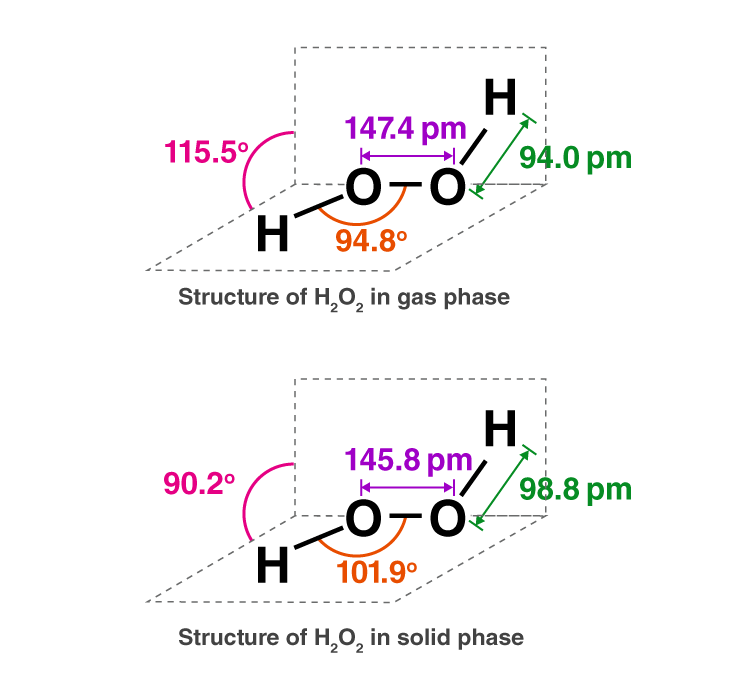(ii) The oxidising state of the oxygen atom on H2O2 is -1 which is an intermediate state. It means that it can reduce itself to H2O where the oxidation state of O is -2. So here, H2O2 acts as an oxidising agent so we can say that H2O2 is a better oxidising agent than water.

41. Melting point, enthalpy of vapourisation and viscosity data of H2O and D2O is

given below :

 Melting point / K Enthalpy of vapourisation at (373 K)/ kJ mol–1 Viscosity/centipoise H2O 373.0 40.66 0.8903 D2O 374.4 41.61 1.107

Based on this data explain in which of these liquids intermolecular

forces are stronger?

Solution:

The values corresponding to the properties are higher for D2O than that of H2O. So, we can say that the intermolecular forces in D2O are stronger.

42. Dihydrogen reacts with dioxygen (O2) to form water. Write the name and formula of the product when the isotope of hydrogen which has one proton and one neutron in its nucleus is treated with oxygen. Will the reactivity of both the isotopes be the same towards oxygen? Justify your answer.

Solution:

Hydrogen has three isotopes – Protium (1H1), Deuterium (2H1), Tritium (3H1). The number of neutrons is the reason why these isotopes differ from each other despite having the same atomic number.

When deuterium reacts with oxygen, deuterium oxide which is heavy water (D2O), is produced.

The D – D bond is stronger than H-H bond, therefore, H2 is more reactive than D2 towards reaction with oxygen. So, they would not have the same reactivity.

43. Explain why HCl is a gas and HF is a liquid.

Solution:

Due to the formation of strong and stable hydrogen bonding, high energy is required to break the H-F bond. So the boiling point of HF is more than HCl and due to which HF is a liquid and HCl is a gas.

44. When the first element of the periodic table is treated with dioxygen, it gives a compound whose solid-state floats on its liquid state. This compound can act as an acid as well as a base. What products will be formed when this compound undergoes authorisation?

Solution:

Hydrogen is the first element and when it reacts with dioxygen it forms water. Water has an amphoteric nature that acts as both acid and base. The solid-state of water is ice. It floats on water due to its lower density.

Auto protolysis or auto ionisation of water is self ionisation of water. It is one of the chemical properties of water. Here, two same molecules react to give ions involving proton transfer. This reaction also shows amphoteric nature.

45. Rohan heard that instructions were given to the laboratory attendant to store a particular chemical i.e., keep it in the darkroom, add some urea in it, and keep it away from dust. This chemical acts as an oxidising as well as a reducing agent in both acidic and alkaline media. This chemical is important for use in the pollution control treatment of domestic and industrial effluents

(i) Write the name of this compound.

(ii) Explain why such precautions are taken for storing this chemical.

Solution:

(i) Hydrogen peroxide is the compound which acts as both oxidizing and reducing agent In acidic and alkali media.

(ii) H2O2 may undergo decomposition into H2Oif it is placed in contact with sunlight or with dust particles. So it is kept in wax-lined coloured bottles.

46. Give reasons why hydrogen resembles alkali metals?

Solution:

Hydrogen is an analogous element as its properties are shared with alkali and halogens in its electronic configuration. The electronic configuration of hydrogen (1s1) is similar to that of alkali metals (ns1) which shows that only one electron is present in their valence shell. So if they lose that single electron, a uni-positive ion would be formed.

47. Hydrogen generally forms covalent compounds. Give reason.

Solution:

Hydrogen can lose or gain an electron to attain noble gas configuration as it has only one electron in its outermost shell. The ionization enthalpy of hydrogen is high and their electron gain enthalpy is slightly negative. So they generally form covalent compounds.

48. Why is the Ionisation enthalpy of hydrogen higher than that of sodium?

Solution:

To remove a valence electron from the H atom is more difficult than Na atom due to higher nuclear attraction so more energy would be required. Thus, ionisation enthalpy of hydrogen is higher than sodium.

49. The basic principle of a hydrogen economy is transportation and storage of energy in the form of liquid or gaseous hydrogen. Which property of hydrogen may be useful for this purpose? Support your answer with the chemical equation if required.

Solution:

Being a liquid, less consumption of volume takes place, so transportation can be done easily. Thus, the basic property of hydrogen which is useful for hydrogen economy is that it can be converted into a liquid – by cooling under high pressure.

50. What is the importance of heavy water?

Solution:

Heavy water is water that contains heavy hydrogen D2O. It is used as a moderator in nuclear reactors and exchange reactions for the study of reaction mechanisms.

51. Write the Lewis structure of hydrogen peroxide.

Solution: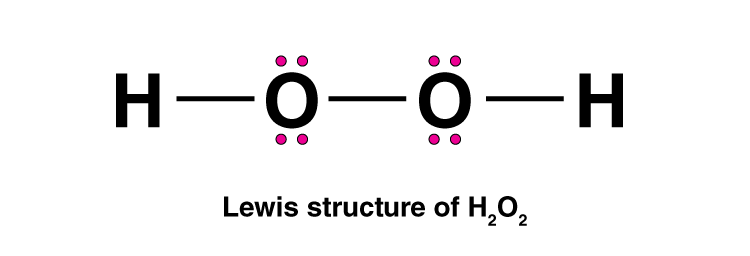52. An acidic solution of hydrogen peroxide behaves as an oxidising as well as the reducing agent. Illustrate it with the help of a chemical equation.

Solution:

H2O2 + 2HI → I2 + 2H2O

Here H2O2 cat as an oxidizing agent

HOCl + H2O2 → H3O+ + Cl + O2

Here H2O2 act as reducing agent

o, we can see that H2O2 acts as an oxidising agent in the first reaction as it increases the oxidation number of I- in HI from -1 to 0 in I2.

Similarly, H2O2 acts as a reducing agent in the second reaction as it decreases the oxidation number of Cl+ in HOCl from +1 to -1 in Cl-.

53. With the help of suitable examples, explain the property of H2O2 that is responsible for its bleaching action?

Solution:

H2O2 or hydrogen peroxide acts as a strong oxidizing agent both in acidic and basic media. It acts as a bleaching agent due to the release of nascent oxygen.

H2O2 ⇌ H2O + O

he nascent oxygen combines with colouring matter breaks the chemical bonds present, which in turn gets oxidised. Hence, the visible light is not absorbed and the cloth gets whitened.

54. Why is water molecule polar?

Solution:

A polar molecule is a molecule that has a slight δ positive and a slight δ negative charge distribution. Due to the high electronegativity difference between oxygen and hydrogen atoms, polarity increases.

55. Why does the water show a high boiling point as compared to hydrogen sulphide? Give reasons for your answer.

Solution:

This is due to the formation of very stable intermolecular hydrogen bonding(bonding with other molecules of the same or different species) because of the presence of electronegative elements like oxygen, fluorine, nitrogen.

#### Important topics of Class 11 Chemistry Chapter 9 Hydrogen

1. Position Of Hydrogen In The Periodic Table
2. Dihydrogen, H2
1. Occurrence
2. Isotopes Of Hydrogen
3. Preparation Of Dihydrogen, H2
1. Laboratory Preparation Of Dihydrogen
2. Commercial Production Of Dihydrogen
4. Properties Of Dihydrogen
1. Physical Properties
2. Chemical Properties
3. Uses Of Dihydrogen
5. Hydrides
1. Ionic Or Saline Hydrides
2. Covalent Or Molecular Hydride
3. Metallic Or Non-stoichiometric (Or Interstitial ) Hydrides
6. Water Ex
1. Physical Properties Of Water Ex
2. Structure Of Water
3. Structure Of Ice
4. Chemical Properties Of Water
5. Hard And Soft Water
6. Temporary Hardness
7. Permanent Hardness
7. Hydrogen Peroxide (H202)
1. Preparation
2. Physical Properties
3. Structure
4. Chemical Properties
5. Storage
6. Uses
8. Heavy Water, D20
9. Dihydrogen As A Fuel.

BYJU’S is dedicated to providing you with the best study material to help you in preparation of CBSE Class 11 examination and entrance examination. You can get notes, study materials, videos animations, exercises, worksheets, previous year question papers, sample papers, books and mock test.

## Frequently Asked Questions on NCERT Exemplar Solutions for Class 11 Chemistry Chapter 9

### What can I learn from the Chapter 9 of NCERT Exemplar Solutions for Class 11 Chemistry?

From the Chapter 9 of NCERT Exemplar Solutions for Class 11 Chemistry, you can learn about topics/subtopics like –
1. Position Of Hydrogen In The Periodic Table
2. Dihydrogen,
Occurrence
Isotopes Of Hydrogen
3. Preparation Of Dihydrogen,
Laboratory Preparation Of Dihydrogen
Commercial Production Of Dihydrogen
4. Properties Of Dihydrogen
Physical Properties
Chemical Properties
Uses Of Dihydrogen
5. Hydrides
Ionic Or Saline Hydrides
Covalent Or Molecular Hydride
Metallic Or Non-stoichiometric (Or Interstitial ) Hydrides
6. Water
Physical Properties Of Water
Structure Of Water
Structure Of Ice
Chemical Properties Of Water
Hard And Soft Water
Temporary Hardness
Permanent Hardness
7. Hydrogen Peroxide
Preparation
Physical Properties
Structure
Chemical Properties
Storage
Uses
8. Heavy Water
9. Dihydrogen As A Fuel.

### How to prepare the Chapter 9 of NCERT Exemplar Solutions for Class 11 Chemistry for board exams?

Students should make use of proper study material while preparing the Chapter 9 of Class 11 Chemistry. The chapter should be revised more than three times to get a clear view about the concepts and the method of solving numericals. By referring to the solutions from BYJU’S, students will be able to get their doubts clarified instantly. It will also improve problem solving and logical thinking skills among students which will help them to perform well in the board exams. The solutions are designed by the experts having conceptual knowledge about the fundamental topics to help students score well in the Class 11 exam.

### How to answer the questions from the Chapter 9 of NCERT Exemplar Solutions for Class 11 Chemistry?

The Chapter 9 of NCERT Exemplar Solutions for Class 11 Chemistry basically has questions related to hydrogen, its preparation and applications. The other important concept is laboratory preparation of dihydrogen. If you are worried about the perfect study material to score more marks in the Class 11 exam, the NCERT Exemplar Solutions at BYJU’S is the best option. The solutions are created in an interactive manner to make learning fun for the students.